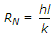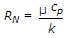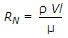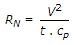# Mechanical Engineering - Heat Transfer, Refrigeration and Air Conditioning - Discussion

19.

Reynolds number (RN) is given by (where h = Film coefficient, l = Linear dimension, V = Velocity of fluid, k = Thermal conductivity, t = Temperature, ρ = Density of fluid, cp = Specific heat at constant pressure, and μ = Coefficient of absolute viscosity)

 [A].[B].[C].[D].Explanation:

No answer description available for this question.

 Rakesh Kumar said: (Jun 20, 2014) Reynolds no is the ratio of inertia force to the viscous force answer C is correct.